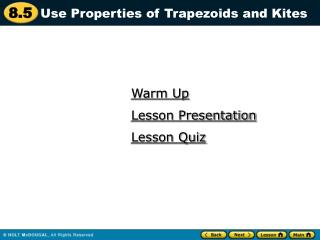DownloadDownload PresentationWarm Up

Warm Up

Télécharger la présentationWarm Up

- - - - - - - - - - - - - - - - - - - - - - - - - - - E N D - - - - - - - - - - - - - - - - - - - - - - - - - - -
Presentation Transcript

1. Use Properties of Trapezoids and Kites Warm Up Lesson Presentation Lesson Quiz

2. ANSWER 125, 125 2. IfAXandBYintersect at pointP,what kind of triangle isXPY? ANSWER isosceles Warm-Up Use the figure to answer the questions. 1. What are the values of xandy?

3. = = Slope of RS = 4 – 3 2 – 0 Slope of OT = = 2 – 0 4 – 0 The slopes of RSand OTare the same, so RSOT . 2 1 1 2 4 2 Example 1 Show that ORSTis a trapezoid. SOLUTION Compare the slopes of opposite sides.

4. –2 –1 = = Slope of ST = 2 , which is undefined = Slope of OR = The slopes of ST and ORare not the same, soST is not parallel to OR . 2 – 4 3 – 0 ANSWER 4 – 2 0 – 0 Because quadrilateral ORST has exactly one pair of parallel sides, it is a trapezoid. 3 0 Example 1

5. 2. In Example 1, which of the interior angles of quadrilateral ORSTare supplementary angles? Explain your reasoning. Parallelogram; opposite pairs of sides are parallel. ANSWER O and R , T and S;Consecutive Interior Angles Theorem ANSWER Guided Practice 1. WHAT IF?In Example 1, suppose the coordinates of point Sare (4, 5). What type of quadrilateral is ORST? Explain.

6. Arch The stone above the arch in the diagram is an isosceles trapezoid. Find mK, mM, and mJ. STEP 1 Find mK.JKLMis an isosceles trapezoid, so Kand Lare congruent base angles, and mK = mL= 85°. Example 2 SOLUTION

7. Find m M. Because Land M are consecutive interior angles formed by LMintersecting two parallel lines, they are supplementary. So, mM = 180° – 85° = 95°. STEP 3 Find mJ. Because J and M are a pair of base angles, they are congruent, and mJ = mM= 95°. ANSWER So, mJ = 95°, m K = 85°, and m M = 95°. Example 2 STEP 2

8. In the diagram,MNis the midsegment of trapezoidPQRS. FindMN. SOLUTION Use Theorem 8.17 to findMN. 1 =(12+ 28) 2 1 MN (PQ + SR) = 2 Example 3 Apply Theorem 8.17. Substitute 12 for PQand 28 for XU. = 20 Simplify. The length MNis 20 inches.

9. ANSWER yes, Theorem 8.16 Guided Practice In Exercises 3 and 4, use the diagram of trapezoidEFGH. 3. If EG = FH, is trapezoid EFGHisosceles? Explain.

10. 4. If mHEF = 70o and mFGH =110o, is trapezoid EFGHisosceles? Explain. SAMPLE ANSWER Yes; mEFG =70° by Consecutive Interior Angles Theorem making EFGH an isosceles trapezoidby Theorem 8.15. Guided Practice In Exercises 3 and 4, use the diagram of trapezoidEFGH.

11. 5. In trapezoid JKLM, Jand M are right angles, and JK = 9 cm. The length of the midsegment NPof trapezoid JKLMis 12 cm. Sketch trapezoid JKLMand its midsegment. Find ML. Explain your reasoning. J K 9 cm ANSWER 12 cm P N M L 1 2 15 cm; Solve for x to find ML. ( 9 + x ) = 12 Guided Practice

12. By Theorem 8.19, DEFGhas exactly one pair of congruent opposite angles. Because E G,Dand Fmust be congruent. So, m D = mF. Write and solve an equation to find mD. Example 4 Find m Din the kite shown at the right. SOLUTION

13. mD + m F + 124° + 80° = 360° mD + m D + 124° + 80° = 360° 2(m D)+ 204° = 360° m D = 78° Substitute m Dfor m F. Solve for m D. Example 4 Corollary to Theorem 8.1 Combine like terms.

14. 25; 75° ANSWER Guided Practice 6. In a kite, the measures of the angles are 3x°, 75°,90°, and 120°. Find the value of x. What are the measures of the angles that are congruent?

15. 1. Find m A, m C, m D. 124°, 56°, 124° ANSWER Lesson Quiz

16. 2. Find the length of the midsegment of the trapezoid. 25 ANSWER Lesson Quiz

17. 3. If m XYZ = 80° and m XWZ=48°, find m YZW. 10 116° 2 , ANSWER ANSWER 2 17 Lesson Quiz Use the figure to find the indicated measures. 4. If XO = 2,OZ = 2, YO = 6, and OW = 8, find the lengths of the sides of the kite.